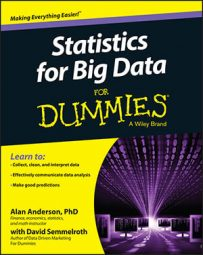##### Statistics for Big Data For DummiesThe two basic types of probability distributions are known as discrete and continuous. Discrete distributions describe the properties of a random variable for which every individual outcome is assigned a positive probability.

A random variable is actually a function; it assigns numerical values to the outcomes of a random process.

Continuous distributions describe the properties of a random variable for which individual probabilities equal zero. Positive probabilities can only be assigned to ranges of values, or intervals. Two of the most widely used discrete distributions are the binomial and the Poisson.

You use the binomial distribution when a random process consists of a sequence of independent trials, each of which has only two possible outcomes. The probabilities of these outcomes are constant on each trial. For example, you could use the binomial distribution to determine the probability that a specified number of defaults will take place in a portfolio of bonds (if you can assume that the bonds are independent of each other).

You use the Poisson distribution when a random process consists of events occurring over a given interval of time. For example, you could use the Poisson distribution to determine the likelihood that three stocks in an investor’s portfolio pay dividends over the coming year.

Some of the most widely used continuous probability distributions are the:

• Normal distribution

• Student’s t-distribution

• Lognormal distribution

• Chi-square distribution

• F-distribution

The normal distribution is one of the most widely used distributions in many disciplines, including economics, finance, biology, physics, psychology, and sociology. The normal distribution is often illustrated as a bell-shaped curve, or bell curve, which indicates that the distribution is symmetrical about its mean. Further, it is defined for all values from negative infinity to positive infinity. Many real-world variables seem to follow the normal distribution (at least approximately), which accounts for its popularity. For example, it’s often assumed that returns to financial assets are normally distributed (although this isn’t entirely correct).

For situations in which the normal distribution is not appropriate, the Student’s t-distribution is often used in its place. The Student’s t-distribution shares several similar properties with the normal distribution; however, the most important difference is that it is more “spread out” about the mean. The Student’s t-distribution is often used for analyzing the properties of small samples.

The lognormal distribution is closely related to the normal distribution, as follows:

• If Y = lnX and X is lognormally distributed, then Y is normally distributed.

• If X = eY and Y is normally distributed, then X is lognormally distributed.

For example, if returns to financial assets are normally distributed, then their prices are lognormally distributed.

Unlike the normal distribution, the lognormal distribution is only defined for non-negative values. Instead of being symmetrical, the lognormal distribution is positively skewed.

The chi-square distribution is characterized by degrees of freedom and is defined only for non-negative values. It is also positively skewed. You can use the chi-square distribution for several applications, including these:

• Testing hypotheses about the variance of a population

• Testing whether a population follows a specified probability distribution

• Determining if two populations are independent of each other

The F-distribution is characterized by two different degrees of freedom: numerator and denominator. It’s defined only for non-negative values and is positively skewed. You can use the F-distribution to determine whether the variances of two populations are equal. You can also use it in regression analysis to determine if a group of slope coefficients are statistically significant.